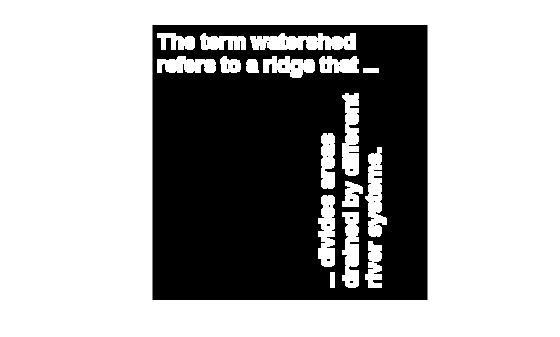# bwunpack

Unpack binary image

## Syntax

``BW = bwunpack(BWP,m)``

## Description

example

````BW = bwunpack(BWP,m)` unpacks the packed binary image `BWP` into binary image `BW` with `m` rows.```

## Examples

collapse all

Read binary image into the workspace.

```BW = imread('text.png'); imshow(BW)```Pack the image.

`BWp = bwpack(BW);`

Dilate the packed image.

`BWp_dilated = imdilate(BWp,ones(3,3),'ispacked');`

Unpack the dilated image and display it.

```BW_dilated = bwunpack(BWp_dilated, size(BW,1)); imshow(BW_dilated)```## Input Arguments

collapse all

Packed binary image, specified as a 2-D numeric array of data type `uint32`.

Data Types: `uint32`

Number of image rows, specified as a positive integer. The default value of `m` is `32*size(BWP,1)`.

Data Types: `uint32`

## Output Arguments

collapse all

Unpacked binary image, returned as a logical matrix with `m` rows.

Data Types: `logical`

## Algorithms

When `bwunpack` unpacks `BWP`, the function maps the least significant bit of the first row of `BWP` to the first pixel in the first row of `BW`. The most significant bit of the first element of `BWP` maps to the first pixel in the 32nd row of `BW`, and so on.

## Version History

Introduced before R2006a